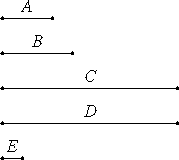# Proposition 16

If two numbers multiplied by one another make certain numbers, then the numbers so produced equal one another.

Let A and B be two numbers, and let A multiplied by B make C, and B multiplied by A make D.

I say that C equals D.VII.Def.15

Since A multiplied by B makes C, therefore B measures C according to the units in A.

But the unit E also measures the number A according to the units in it, therefore the unit E measures A the same number of times that B measures C.

VII.15

Therefore, alternately, the unit E measures the number B the same number of times that A measures C.

Again, since B multiplied by A makes D, therefore A measures D according to the units in B. But the unit E also measures B according to the units in it, therefore the unit E measures the number B the same number of times that A measures D.

But the unit E measures the number B the same number of times that A measures C, therefore A measures each of the numbers C and D the same number of times.

Therefore C equals D.

Therefore, if two numbers multiplied by one another make certain numbers, then the numbers so produced equal one another.

Q.E.D.

## Guide

This proposition states the commutativity of multiplication of formal numbers, ab = ba.

#### Outline of the proof

Let a = nu and b = mu. Then by the definition of multiplication of numbers, ab = nb, and ba = ma. By the preceding proposition, n(mu) = m(nu). Therefore, ab = ba.

#### Use of Proposition 16

This proposition is used in VII.18 and a few others in Book VII.